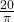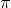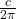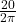## My circumference is 20 cm what is my diameter and radius?

Question

My circumference is 20 cm what is my diameter and radius?

in progress 0
5 months 2021-08-29T12:15:32+00:00 1 Answers 3 views 0

The diameter of the circle would be: 6.4 cm. rounded or 6.36619772 cm.

The radius of the circle would be: 3.2 cm. rounded or 3.18309886 cm.

Step-by-step explanation:

### The diameter can be solved using: D =So, the first step would be : D =Then : D = 20 ÷Finally, the answer will be : 6.36619772 cm. or 6.4 cm.

### The Radius can be Solved using: r =The first step would be : r =Then : 20 ÷ 6.2831853071796 (2)

Finally, the answer will be : 3.18309886 cm. or 3.2 cm.